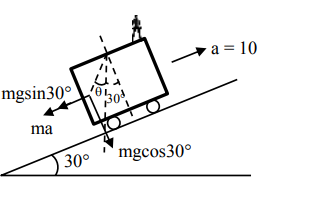# A car is moving on a plane inclined at`
Question:

A car is moving on a plane inclined at $30^{\circ}$ to the horizontal with an acceleration of $10 \mathrm{~ms}^{-2}$ parallel to the plane upward. A bob is suspended by a string from the roof of the car.The angle in degrees which the string makes with the vertical is (Take $\mathrm{g}=10 \mathrm{~ms}^{-2}$ )

Solution:$\tan (30+\theta)=\frac{m g \sin 30^{\circ}+m a}{m g \cos 30^{\circ}}$

$\tan (30+\theta)=\frac{5+10}{5 \sqrt{3}}=\frac{1+2}{\sqrt{3}}$

$\frac{\tan \theta+\frac{1}{\sqrt{3}}}{1-\frac{1}{\sqrt{3}} \tan \theta}=\sqrt{3}$

$\sqrt{3} \tan \theta+1=3-\sqrt{3} \tan \theta$

$2 \sqrt{3} \tan \theta=2$

$\tan \theta=\frac{1}{\sqrt{3}}$

$\theta=30^{\circ}$